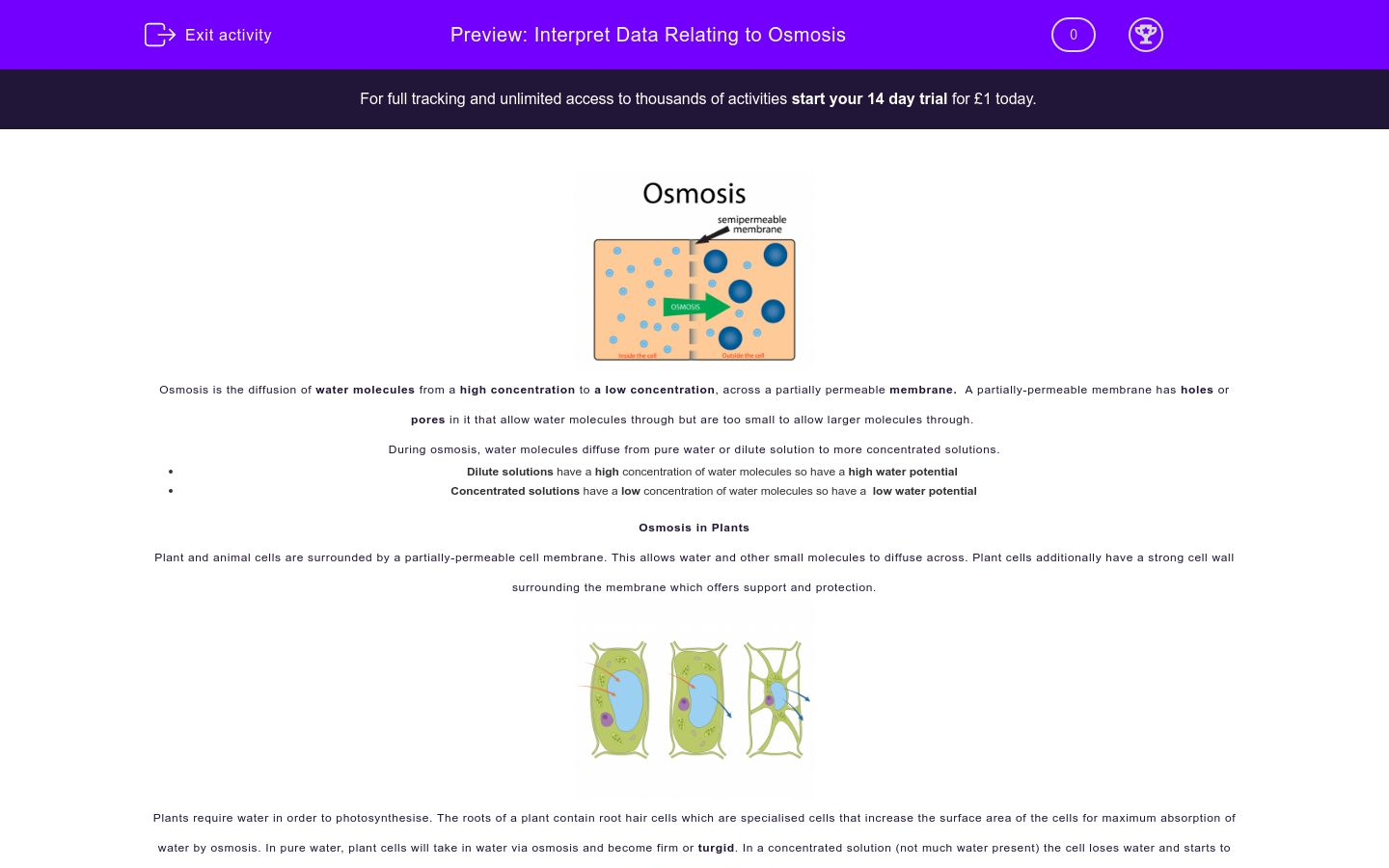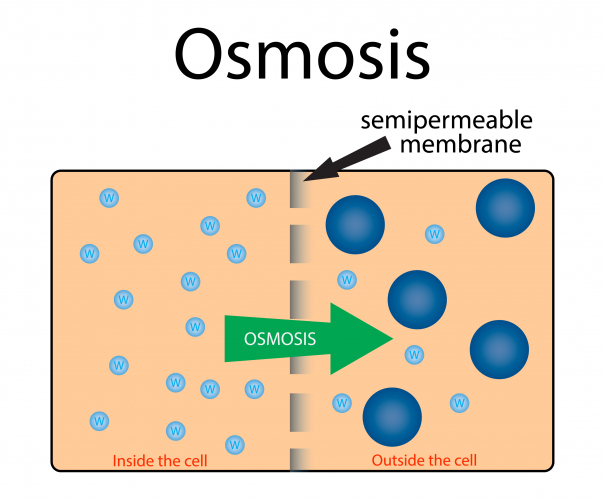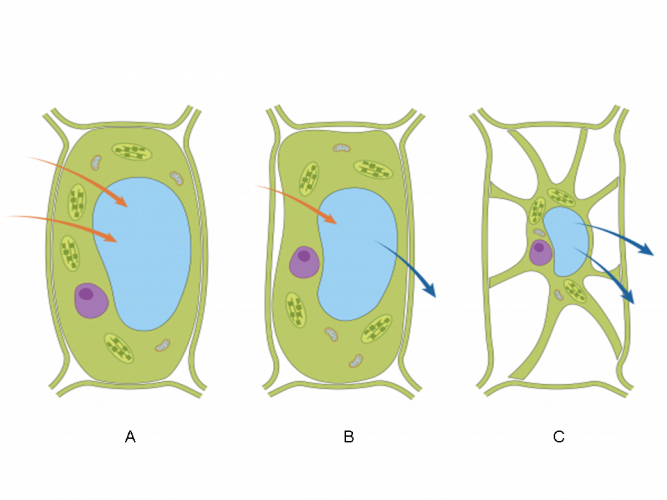# Interpret Data Relating to Osmosis

In this worksheet, students will interpret data relating to osmosis.Key stage:  KS 4

GCSE Subjects:   Biology: Single Subject, Biology: Combined Science

GCSE Boards:   OCR 21st Century

Curriculum topic:   Living Together: Food and the Ecosystem

Difficulty level:### QUESTION 1 of 10Osmosis is the diffusion of water molecules from a high concentration to a low concentration, across a partially permeable membrane.  A partially permeable membrane has holes or pores in it that allow water molecules through, but are too small to allow larger molecules through.

During osmosis, water molecules diffuse from pure water or dilute solution to more concentrated solutions.

Dilute solutions have a high concentration of water molecules, so have a high water potential.

Concentrated solutions have a low concentration of water molecules, so have a  low water potential.

Osmosis in Plants

Plant and animal cells are surrounded by a partially permeable cell membrane. This allows water and other small molecules to diffuse across. Plant cells additionally have a strong cell wall surrounding the membrane, which offers support and protection.Plants require water in order to photosynthesise. The roots of a plant contain root hair cells, which are specialised cells that increase the surface area of the cells for maximum absorption of water by osmosis. In pure water, plant cells will take in water via osmosis and become firm or turgid. In a concentrated solution (not much water present), the cell loses water and starts to shrink and becomes flaccid.

In humans, the concentration of water and salt in the blood is controlled by the kidneys. The kidneys ensure our bodies have the right concentration of water by getting rid of the excess water as urine.

Calculations Involving OsmosisOsmosis can be demonstrated using cubes of potatoes of roughly the same mass. By placing the cubes in different concentrations of sugar solutions, the cubes might gain or lose mass, or might even stay the same mass.

Scientists will be able to calculate the percentage change in mass to see how much mass was gained or lost - and converting to percentages often makes for easier comparison. Percentage change in mass is calculated by using the following equation:

(Final mass (g) – initial mass (g)) ÷ initial mass (g) x 100

For example, a potato cube has an initial mass of 1 g. After placing it in a sugar solution for 30 minutes, its mass was 1.1 g. Its percentage change in mass is 10%.

(1.1 g - 1 g) ÷ 1 g x 100 = +10%

The plus sign shows there was an increase in mass. A minus sign shows a loss in mass.

The rate of water uptake can also be calculated. This gives an indication of how much water is absorbed in an hour. The rate of uptake is calculated using the following equation:

Change in mass (g) x (60 minutes ÷ duration of time in minutes)

Using the above example of the potato cube:

0.1 g x (60 mins ÷ 30 mins) = 0.2 g/hour

In the following activity, you will interpret data relating to osmosis.

Root hair cells use the process of osmosis to transport water into or out of their cells.

Describe osmosis.

.Lara did an experiment at school on the effect of sugar solutions on potato pieces.  The potato pieces were of equal size. She measured the mass of each potato cube and placed each one in a beaker containing different concentrations of sugar solution. After two hours, each potato cube's mass was measured.

 Beaker 1 2 3 4 5 Sugar concentration (M) 0.2 0.4 0.6 0.8 1 Initial mass (g) 2 2 2 2 2 Final mass (g) 2.2 2.15 1.96 1.8 1.73

Calculate the change in mass in beaker 2 and beaker 3.

Lara did an experiment at school on the effect of sugar solutions on potato pieces.  The potato pieces were of equal size. She measured the mass of each potato cube and placed each one in a beaker containing different concentrations of sugar solution. After two hours, each potato cube's mass was measured.

 Beaker 1 2 3 4 5 Sugar concentration (M) 0.2 0.4 0.6 0.8 1 Initial mass (g) 2 2 2 2 2 Final mass (g) 2.20 2.15 1.96 1.8 1.73 % change in mass (a) 7.5 -2 -10 (b)

Calculate the percentage change in mass in beaker 1 (a) and beaker 5 (b).

(a) 10%

(b) -8%

(a) -10%

(b) -13.5%

Lara did an experiment at school on the effect of sugar solutions on potato pieces.  The potato pieces were of equal size. She measured the mass of each potato cube and placed each one in a beaker containing different concentrations of sugar solution. After two hours, the mass of each potato cube was measured.

 Beaker 1 2 3 4 5 Sugar concentration (M) 0.2 0.4 0.6 0.8 1 Initial mass (g) 2 2 2 2 2 Final mass (g) 2.2 2.15 1.96 1.8 1.73 % change in mass 10 7.5 -2 -10 -13.5

Explain why the mass decreased in the potatoes in beakers 3, 4 and 5.

Water entered the potato for respiration

Water left the potato cells by osmosis

The concentration of water outside the cells was higher than inside the cells

The concentration of water outside the cells was lower than inside the cellsCell A < Permeable membraneCell B

The diagram above shows two cells separated by a permeable membrane. Cell A and Cell B both have twelve molecules of water.

In which direction will the water move?

From Cell A to Cell B

From Cell B to Cell A

No net movement

Plant cell membranes are partially permeable.

What is a partially permeable membrane?A partially permeable membrane does not have holes in it

A partially permeable membrane has holes in it

It only allows waste products through

It allows water molecules through but the holes are too small to allow larger molecules through

Lara did an experiment at school on the effect of sugar solutions on potato pieces.  The potato pieces were of equal size. She measured the mass of each potato cube and placed each one in a beaker containing different concentrations of sugar solution. After 50 minutes, each potato cube's mass was measured.

 Beaker 1 2 3 4 5 Sugar concentration (M) 0.2 0.4 0.6 0.8 1 Initial mass (g) 2 2 2 2 2 Final mass (g) 2.2 2.15 1.96 1.8 1.73 % change in mass 10 7.5 -2 -10 -13.5

Calculate the rate of uptake in an hour for beaker 2.

0.24

0.20

0.18

Plants often lose water from the stomata found on the underside of their leaves. The image below is of a cell from a plant in hot and dry conditions.

What is happening to the water in this cell?0.24

0.20

0.18

Match up the plant cells in the picture below with the type of solutions they are in.Plant cells will respond in different ways to the presence or absence of water.

Compare the plant cells in the image below by matching the cells to the descriptions.## Column B

Cell A
Cell becomes flaccid, the solution is concentrated...
Cell B
Water moves into and out of the cell by osmosis at...
Cell C
Cell becomes turgid, the solution is dilute so wat...
• Question 1

Root hair cells use the process of osmosis to transport water into or out of their cells.

Describe osmosis.

.EDDIE SAYS
Did you remember the definition? It is vital that you remember which way the water molecules move in osmosis - from a high concentration of water molecules (that is a diluted solution) to a low concentration of water (that is a concentrated solution). If you're finding this tricky, why not read the Introduction again before moving on with the questions. Osmosis is really important for cells, they're able to get the water they need for different processes like photosynthesis.
• Question 2

Lara did an experiment at school on the effect of sugar solutions on potato pieces.  The potato pieces were of equal size. She measured the mass of each potato cube and placed each one in a beaker containing different concentrations of sugar solution. After two hours, each potato cube's mass was measured.

 Beaker 1 2 3 4 5 Sugar concentration (M) 0.2 0.4 0.6 0.8 1 Initial mass (g) 2 2 2 2 2 Final mass (g) 2.2 2.15 1.96 1.8 1.73

Calculate the change in mass in beaker 2 and beaker 3.

EDDIE SAYS
How did you get on with this maths question? It's not too tricky so as long as you can remember the formula! To calculate the change in mass: Final mass – initial mass For Beaker 2: 2.15 - 2 = 0.15 g For Beaker 3: 1.96 - 2 = -0.04 g Just make sure you learn the necessary formulas for these questions and you're halfway there.
• Question 3

Lara did an experiment at school on the effect of sugar solutions on potato pieces.  The potato pieces were of equal size. She measured the mass of each potato cube and placed each one in a beaker containing different concentrations of sugar solution. After two hours, each potato cube's mass was measured.

 Beaker 1 2 3 4 5 Sugar concentration (M) 0.2 0.4 0.6 0.8 1 Initial mass (g) 2 2 2 2 2 Final mass (g) 2.20 2.15 1.96 1.8 1.73 % change in mass (a) 7.5 -2 -10 (b)

Calculate the percentage change in mass in beaker 1 (a) and beaker 5 (b).

(a) 10%
(b) -13.5%
EDDIE SAYS
How are you doing with these maths questions? What is the formula you need this time? To calculate the percentage change in mass: (Final mass – initial mass) ÷ initial mass x 100 Use BIDMAS to get the order correct. Beaker 1: (2.20 - 2) ÷ 2 x 100 Brackets first: 2.20 - 2 = 0.20 Division next: 0.20 ÷ 2 = 0.1 Finally, multiply: 0.1 x 100 = 10% Beaker 5: (1.73 - 2) ÷ 2 x 100 Brackets: 1.73 - 2 = -0.27 Divide: -0.27 ÷ 2 = -0.135 Multiply: -0.135 x 100 = -13.5% Just remember to use the BIDMAS order and you're there!
• Question 4

Lara did an experiment at school on the effect of sugar solutions on potato pieces.  The potato pieces were of equal size. She measured the mass of each potato cube and placed each one in a beaker containing different concentrations of sugar solution. After two hours, the mass of each potato cube was measured.

 Beaker 1 2 3 4 5 Sugar concentration (M) 0.2 0.4 0.6 0.8 1 Initial mass (g) 2 2 2 2 2 Final mass (g) 2.2 2.15 1.96 1.8 1.73 % change in mass 10 7.5 -2 -10 -13.5

Explain why the mass decreased in the potatoes in beakers 3, 4 and 5.

Water left the potato cells by osmosis
The concentration of water outside the cells was lower than inside the cells
EDDIE SAYS
Did you get this one right? Remember which way water moves in osmosis? Water moves from where it is more concentrated to where it is less concentrated. In beakers 3, 4 and 5, the concentration of sugar is high, meaning that the concentration of water will be lower in the solution than in the cells of the potatoes. This means that the water will move out of the potatoes, resulting in a decrease in mass.
• Question 5Cell A < Permeable membraneCell B

The diagram above shows two cells separated by a permeable membrane. Cell A and Cell B both have twelve molecules of water.

In which direction will the water move?

No net movement
EDDIE SAYS
This is common sense really if you think about it. There are equal numbers of water molecules in Cell A and Cell B, so water will continue to move at an equal rate between the cells.
• Question 6

Plant cell membranes are partially permeable.

What is a partially permeable membrane?A partially permeable membrane has holes in it
It allows water molecules through but the holes are too small to allow larger molecules through
EDDIE SAYS
A rather more straightforward question this time. A partially permeable membrane is selective and only allows water and small particles to pass through it.
• Question 7

Lara did an experiment at school on the effect of sugar solutions on potato pieces.  The potato pieces were of equal size. She measured the mass of each potato cube and placed each one in a beaker containing different concentrations of sugar solution. After 50 minutes, each potato cube's mass was measured.

 Beaker 1 2 3 4 5 Sugar concentration (M) 0.2 0.4 0.6 0.8 1 Initial mass (g) 2 2 2 2 2 Final mass (g) 2.2 2.15 1.96 1.8 1.73 % change in mass 10 7.5 -2 -10 -13.5

Calculate the rate of uptake in an hour for beaker 2.

0.18
EDDIE SAYS
Another maths question to do here. Can you remember the formula to use? To calculate the rate of uptake: Change in mass x (60 minutes ÷ time measured in minutes) The change of mass in beaker 2 is 2.15 - 2 = 0.15 0.15 x (60 ÷ 50) Brackets first: 60 ÷ 50 = 1.2 0.15 x 1.2 = 0.18 It's quite simple so long as you remember the formula and use BIDMAS to get the correct order!
• Question 8

Plants often lose water from the stomata found on the underside of their leaves. The image below is of a cell from a plant in hot and dry conditions.

What is happening to the water in this cell?EDDIE SAYS
Did you fill in all three blanks correctly? Don't worry if you put something else for the final blank - just try to remember that strange word, flaccid, which is similar to the word floppy. A plant cell that has its cell membrane pulled away from the cell wall is showing a flaccid cell. All the water has left the cell by osmosis. It's a bit like using a straw to suck out all the juice from a carton - the carton loses its shape.
• Question 9

Match up the plant cells in the picture below with the type of solutions they are in.EDDIE SAYS
One final test of your understanding here! How did you get on with it? Remember if you see a swollen plant cell, it's because lots of water has moved into it by osmosis - due to the fact that the solution it is in has a higher water concentration than the cell itself. The opposite is true for a shrivelled looking plant cell where water has moved from the cell (with a high water concentration) to a more concentrated solution (containing less water). With the isotonic solution in cell B, water is moving into and out of the cell at the same rate so there is no overall change. Phew - there was a lot to think about in this activity. Hopefully though, you're feeling more confident with this topic now.
• Question 10

Plant cells will respond in different ways to the presence or absence of water.

Compare the plant cells in the image below by matching the cells to the descriptions.## Column B

Cell A
Cell becomes turgid, the solution...
Cell B
Water moves into and out of the c...
Cell C
Cell becomes flaccid, the solutio...
EDDIE SAYS
This was quite a tricky question - how did you get on with it? Remember that if you see a swollen plant cell, it's because lots of water has moved into it by osmosis. The opposite is true for a shrivelled looking plant cell. In cell A, the solution is dilute so that water molecules move into the cells of the potato, causing it to swell and become turgid. In cell C, the solution is concentrated, which means that water molecules will move out of the cells, making them floppy or flaccid. Cell B shows an isotonic solution where water is moving into and out of the cell at the same rate. This means that the cell doesn't change in size.
---- OR ----